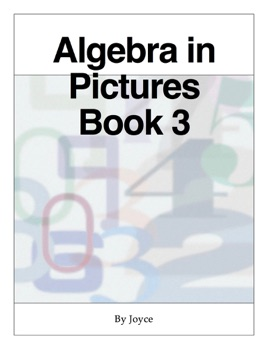## Description de l’éditeur

Algebra in Pictures Book 3 is the ninth book in the series, covering solving simultaneous equations, and cases where there is no solution, or an infinite number of solutions. Results are illustrated by graphs. Solving quadratic equations by factorising, including the special cases of a perfect square, the difference of two squares and no constant term. Completing a square in a quadratic expression by combining unknown terms into a perfect square, applying this method to find maximum and minimum values of a quadratic function. Applying the formula for the general solution of a quadratic equation. Solving simultaneous linear and quadratic equations. Solving simultaneous quadratic and cubic equations by plotting graphs, and using the method of trial & improvement to give solutions to a given degree of accuracy. Looking at linear inequalities by shading regions in a co-ordinate diagram, and showing solutions satisfying three inequalities by shading unwanted regions. Solving simultaneous inequalities by adding and multiplying.

Review questions are at the end of each section.

The review questions require iOS 10 or higher.